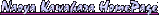# 1\$B?M%Y%s%A\$G;W\$&\$3\$H(B

\$B\$3\$N?'\$O!"F|5-!#(B
\$B\$3\$N?'\$O!"99?75-O?(B\$B!#(B

2001\$BG/(B4\$B7n8eH>(B \$BF|5-%\$%s%G%/%9(B 2001\$BG/(B5\$B7n8eH>(B

\$B\$3\$N(B html \$BFb\$NFCDjJ8>O\$X\$N%j%s%/\$O!"(B
http://www.pluto.dti.ne.jp/~nkf/diary/01may_a.html#010501a
\$B\$J\$I\$KE=\$C\$F2<\$5\$\$!#(B

2001/05/14(mon)
\$B&O(B \$BMU;3\$5\$s(B\$B\$H\$\$\$&?M\$,(B Windows \$B\$G;H\$(\$k7Z\$=\$&\$J%7%'%k\$r:n\$C\$F\$\$\$k\$N\$r?&>l\$GH/8+\$7\$?\$N\$G!"(B \$B\$H\$C\$F\$-\$F\$_\$?!#(B \$B\$7\$+\$7\$3\$s\$J\$H\$3\$m\$K\$b(B saparak \$B\$X\$N%j%s%/\$,\$"\$k\$s\$G\$9\$J!#(B \$B\$F\$\$\$&\$+\$b\$H\$b\$H\$=\$&\$\$\$&4X78\$+!#(B
\$B&O(B \$B\$7\$+\$7(B history-search-backward \$B\$H!"(Bhistory-search-forward \$B\$,M_\$7\$\$\$G\$9\$,(B \$B<+J,\$G:n\$l\$H\$\$\$&@<\$bJ9\$3\$(\$^\$9\$M\$(!D7k9=\$7\$s\$I\$\$\$s\$G\$9\$,!#(B
\$B&O(B \$B\$"\$H(B \$B\$J\$<\$+%a%b%j%W%m%F%/%H\$r(B on \$B\$K\$9\$k\$H%(%i!<\$,\$G\$k\$s\$G\$9\$,!"(B
2001/05/13(sun)
\$B&O(B NTTPHS \$B2rLs\$7\$^\$7\$?!#(B
\$B&O(B 641Sf \$B\$,%*%l\$K\$O9g\$o\$J\$\$\$H\$\$\$&\$N\$H!"(B \$B:#8e\$\$\$\$CuBV\$K\$J\$C\$F\$\$\$?\$s\$G!"(B \$B\$b\$&\$\$\$\$\$+\$H!#(B
\$B&O(B \$B\$=\$l\$G\$b!"Nc\$(\$P(B 60g \$B\$NC.\$5\$\$C.\$5\$\$C
\$B&O(B \$B2rLs\$HF1;~\$K(B P503iS \$B\$rGc\$C\$F\$_\$F\$b\$\$\$\$\$+\$J\$!!A\$J\$s\$F\$\\$s\$d\$j;W\$\$\$J\$,\$i!"(B \$B%I%3%b%7%g%C%W\$K8~\$+\$C\$?\$N\$@\$,!"%b%C%/%"%C%W\$r8+\$F!"(B \$B\$"\$NC
\$B&O(B P209iS \$B\$r:G=i\$K8+\$?\$H\$-\$K!V\$G\$+\$9\$.\$k!#%@%a%@%a!W\$H;W\$C\$??M4V\$K(B \$BGc\$(\$kCfIW\$@!#(B
\$B&O(B \$B\$=\$s\$G(B P158 \$B\$N%b%C%/%"%C%W\$r\$*;}\$A5"\$j!D\$d\$C\$Q\$j(B P208/P158 \$B\$O\$\$\$\$\$M!#(B \$B\$3\$NN"LL\$,\$?\$^\$j\$^\$;\$s\$J!#(B
2001/05/11(fri)
\$B&O(B \$B2q5D\$NM=Dj\$,\$"\$C\$?\$,!"?2K7\$7\$FCY9o\$9\$kL4\$r8+\$F%"%;\$k!#(B
\$B&O(B \$B\$=\$7\$F2q5D!#;`\$L\$M!#(B
2001/05/09(wed)
\$B&O(B \$BF|IU\$,A4It(B2000\$BG/\$@(B\$B\$C\$?\$N\$rD>\$7\$^\$7\$?\$C!#(B \$BF|IU\$r%+%C%H%"%s%I%Z!<%9%H\$GF~NO\$7\$F\$k\$N\$,%P%l\$^\$9\$J!#(B
\$B&O(B \$B:rF|\$N%M%?\$r\$5\$/\$j\$H\$D\$/\$C\$F\$_\$^\$7\$?!#(B \$B\$3\$3(B\$B!#\$@\$+\$i\$J\$s\$@\$H\$\$\$&46\$8\$G\$O\$"\$j\$^\$9!#(B
2001/05/08(tue)
\$B&O(B PSO\$B%;%/%7%g%s(BID \$B7W;;(B\$B!#(B \$B\$3\$3(B\$B\$H\$+(B \$B\$3\$3(B \$B\$H\$+(B \$B\$3\$3(B \$B\$K\$"\$k(B script \$B\$KHf\$Y\$k\$H\$+\$J\$jAGE(\$@!#(B
onKeyUp \$B%\$%Y%s%H\$r;H\$&\$H\$+!"(B \$BI=<(\$9\$k%a%C%;!<%8\$NJ8;zNs\$r\$"\$i\$+\$8\$a@8@.\$7\$F\$*\$\$\$F%\$%Y%s%H%O%s%I%iFb\$G\$O(B \$B\$=\$N%j%U%!%l%s%9\$@\$1;H\$&\$H\$+\$O!"(B \$B\$5\$9\$,%W%m(B(?)\$B\$N;E6H\$G\$"\$k\$H>!
\$B&O(B i \$B%"%W%j\$G:n\$k(B\$B\$H\$\$\$&%M%?\$r9M\$(\$?\$N\$@\$,!":n\$C\$F\$bA0F~NO\$9\$k\$N\$O(B \$BBgJQ\$=\$&\$J\$s\$G
\$B&O(B Libretto L1 \$B\$+!#(BLibretto \$B\$H\$7\$F\$O%@%a\$9\$.!"(BLibretto \$B\$H9M\$(\$J\$1\$l\$P!"\$=\$3\$=\$3\$N%^%7%s!#(B \$B\$@\$,!"\$=\$3\$=\$3\$G\$7\$+\$J\$\$!#\$D\$^\$j%\$%s%Q%/%H\$,Hy?P\$b46\$8\$i\$l\$J\$\$!#(B
\$BB?J,\$3\$N(B Libretto \$B\$O\$=\$3\$=\$3Gd\$l\$k\$@\$m\$&\$1\$I!"(B \$B?7\$7\$\$;H\$\$J}\$O\$3\$N%^%7%s\$+\$i\$O=P\$F\$3\$J\$\$\$N\$@!#(B \$B!V?7(BLibretto\$B\$G\$O!\$K\Mh\$N%3%s%;%W%H\$KN)\$ALa\$j!\$(B \$B;T>l\$,El/\$J\$/\$H\$b(B Libretto \$B\$N%3%s%;%W%H\$C\$F\$=\$s\$J\$D\$^\$s\$J\$\$\$b\$N\$G\$O(B \$B\$J\$+\$C\$?\$O\$:\$@!#\$@\$C\$?\$i!"\$=\$NH/8@\$G8@\$C\$F\$k!VK\Mh\$N%3%s%;%W%H!W\$C\$F\$N\$O(B \$B\$\$\$D\$N%3%s%;%W%H\$N\$3\$H\$J\$s\$@\$m\$&(B?
\$B&O(B \$B\$3\$N%5%\$%:\$@\$H3+H/\$9\$kB&\$O(B \$B!VB>o\$K3Z%A%s\$J\$N\$@!#\$3\$l\$,(B 800g \$B%N!<%H\$@\$H!"(B \$B!V(B(101\$B!A(B103 \$B\$N:"\$N(B) FIVA \$B\$_\$?\$\$\$K!"7k6IGd\$l\$J\$\$\$s\$8\$c\$J\$\$\$N(B?\$B!W\$H\$\$\$&(B \$BFM\$C9~\$_\$KBP\$7\$FAjEv\$K@bF@NO\$N\$"\$kH?O@\$r\$7\$J\$-\$c\$\$\$1\$J\$\$\$s\$@\$m\$&!#(B
\$B\$"\$k0UL#(B Libretto L1 \$B\$O\$=\$&\$\$\$&EXNO\$rJ|4~\$7\$?\$H\$\$\$&\$h\$&\$K\$b8+\$(\$F\$7\$^\$&\$N\$@!#(B
2001/05/07(mon)
\$B&O(B onda\$B\$5\$s\$N\$H\$3\$m(B\$B\$+\$i(B AYBABTU\$B!#(B \$B%`!<%S!<\$,%&%1\$k!#\$"\$H\$O(B \$B%j%s%/\$N?t!9\$K\$S\$C\$/\$j!#(B
"War was beginning."
"What happen?"

\$B\$N;~E@\$G\$+\$J\$j\$*\$+\$7\$\$\$H;W\$&\$7!"J8L.E*\$K\$O(B
"How are you gentlemen!!"

\$B\$,6/Nu\$K\$*\$+\$7\$\$\$N\$@\$1\$I!"J8C1BN\$G\$O(B "All your base are belong to us." \$B\$OFC\$K%D%\\$K\$O\$^\$k\$s\$@\$m\$&\$+!#(B
\$B3N\$+\$K!V\$3\$\$\$s\$\$\$C\$3\$\$\$l\$k!W\$K\$7\$F\$b(B \$B%G%#%C%/%H%l%\$%7!<\$N%^%K%e%"%k(B \$B\$K\$7\$F\$b\$J\$s\$+%X%s\$@\$1\$IJ8K!\$O\$<\$s\$<\$sGKC>\$7\$F\$J\$\$\$+\$i\$J\$"!#(B
\$B&O(B \$B\$7\$+\$7:#\$4\$mEl0!%W%i%s\$,M-L>\$K\$J\$k\$H\$O;W\$o\$J\$+\$C\$?\$J!#(B
2001/05/06(sun)
\$B&O(B \$BK\C*\$rGc\$\$\$K%8%c%9%3\$K!#8aA0Cf\$O\$=\$s\$J\$KCsl\$KF~\$k\$N\$KBT\$?\$5\$l\$J\$\$\$s\$@\$J!#(B
\$B&O(B \$B%8%c%9%3\$O\$\$\$^\$\$\$A\$@\$C\$?\$,!"\$=\$N6a\$/\$K2H6q20\$H%[!<%`%;%s%?!
\$B&O(B \$B%_%I%jEE2=\$O(B CPU \$B%U%!%s\$H\$+(B IDE\$B%1!<%V%k\$H\$+\$\$\$m\$\$\$mGd\$C\$F\$\$\$F\$9\$P\$i\$7\$\$\$G\$9\$J!#(B \$B\$<\$R3hMQ\$7\$F\$\$\$-\$?\$\$!#(B \$B\$7\$+\$7(B\$B%T%C%H%o%sIz8+E9(B \$B\$b%*!<%W%s\$7\$F\$\$\$k\$N\$G!"\$"\$^\$j%_%I%jEE2=\$^\$G9T\$+\$J\$\$\$+\$b\$7\$l\$J\$\$!#(B \$B=jA'6a>l\$K\$=\$l\$J\$j\$NE9\$,\$"\$l\$P6a>l\$G:Q\$^\$;\$F\$7\$^\$&\$b\$N\$J\$N\$@!#(B
\$B&O(B CD\$B\$rBgNL\$K<}G<\$G\$-\$k%U%!%\$%k\$r%2%C%H\$7\$F!"(B \$B%;%,%5%?!<%sMQ%2!<%`\$N(BCD-ROM\$B\$rA4It\$=\$3\$K<}G
\$B&O(B \$B:#F|\$O%9%Q%2%F%#20\$K\$O\$\$\$1\$J\$+\$C\$?\$N\$G!V(B\$BH`;aJg=8Cf(B\$B!W\$N\$*\$M\$\$\$5\$s\$K\$O2q\$(\$:!#(B
\$B&O(B iBook \$B\$NOC!#(B\$B\$3\$N\$H\$-(B\$B\$O(B PBG4 \$B\$H\$N:9\$,\$J\$\$\$J\$"\$H;W\$C\$F\$\$\$?\$,!"(B \$B\$=\$b\$=\$b30Au\$,%]%j%+!<%\%M!<%H\$+6bB0\$+\$C\$F\$\$\$&;~E@\$G8+\$?L\\$N0c\$\$\$O(B \$B7hDjE*\$@\$m\$&!#(B \$B
2001/05/05(sat)
\$B&O(B \$BD+(B9\$B;~\$K?2\$F!"5/\$-\$?\$iLk(B9\$B;~\$@\$C\$?(B \$B\$H\$\$\$&L5BL\$J0lF|\$N2a\$4\$7J}\$r\$9\$k!#(B
2001/05/04(fri)
\$B&O(B \$BIt20\$NA]=|\$r\$7\$F2a\$4\$9!#D+\$+\$i\$9\$4\$/BgNL\$N%4%_\$rl=j\$K:\$\$C\$F\$\$\$k\$N\$G(B \$B%9%?%C%+%V%k\$JCV\$-Bf\$rGc\$C\$F\$-\$F%-!<%\!<%I\$r(B2\$B=E\$K@_CV\$7\$F\$_\$?\$,!"(B \$B8+\$?L\\$,\$+\$C\$3\$o\$k\$9\$.\$?\$N\$G\$d\$a\$?!#(B
\$B&O(B \$B%I%3%b(BPHS\$B\$O2rLs\$9\$kJ}8~\$K?4\$,F0\$-\$D\$D\$"\$k!#(B \$B\$H\$j\$"\$(\$:(B 641Sf \$B\$O;H\$\$\$D\$E\$1\$k5\$\$K\$J\$l\$J\$\$!#(B 621S \$B\$KLa\$7\$F\$b\$\$\$\$\$,!"(B \$B\$=\$l\$@\$HCuBV\$J\$N\$G!"(B \$B2rLs\$7\$F\$bLdBj\$J\$/\$J\$C\$?\$N\$@!#(B
\$B&O(B \$B%a!<%k\$N<+F0FI\$_9~\$_\$,\$J\$/\$J\$C\$?\$N\$ODK\$\$\$G\$9\$h!#(B \$B\$3\$l\$O%5!<%P\$NIi2Y\$NLdBj\$G\$J\$/\$J\$C\$?\$s\$8\$c\$J\$\$\$+\$H5?\$C\$F\$\$\$k\$N\$@\$1\$I!"(B \$B
2001/05/03(thu)
\$B&O(B \$BFy\$/\$\$\$^\$/\$j\$G\$9\$h!#FC\$K7\!#(B
\$B&O(B \$B\$1\$\$\$O\$s\$J5-G08x1`(B\$B\$K\$D\$l\$F\$\$\$C\$F\$b\$i\$C\$F!"(B \$BFf\$NM76q\$GM7\$V!#\$_\$s\$J855\$\$J\$s\$G%*%l\$N\$h\$&\$JG/4s\$j\$O%R%\$\$F\$7\$^\$&\$,!"(B \$B%a%s%P!<\$NCf\$G\$O%*%l\$,0lHV
2001/05/02(wed)
\$B&O(B \$B5/\$-\$F!"(Bapple.co.jp \$B\$X!#\$*\$*!#(B \$B?7(B iBook \$B\$,=P\$F\$k(B\$B\$J!#(B CD-RW \$BFbB"\$G(B 2.2kg \$B\$C\$F\$N\$O0l@NA0\$J\$i9M\$(\$i\$l\$J\$+\$C\$?=E\$5\$J\$o\$1\$G!"(B \$B\$=\$&\$\$\$&0UL#\$G\$O\$A\$c\$s\$H\$,\$s\$P\$C\$F\$\$\$k!#(B \$B1U>=\$,Bg\$-\$/\$J\$C\$F!"%9%T!<%+\$,%9%F%l%*\$K\$J\$C\$F!"(B DVD-ROM/CD-RW \$B%3%s%\%I%i%\$%V\$bEk:\\$G\$-\$k!#(B \$B\$J\$s\$F\$\$\$&\$+(B iBook \$B\$N%@%a\$JE@\$,\$+\$J\$j2~A1\$5\$l\$?!#(B
\$B&O(B \$B7gE@\$O%G%6%\$%s\$@\$m\$&\$J\$"!#(B \$B\$3\$N%G%6%\$%s\$G\$O!"(BPowerBook \$B\$H\$N0c\$\$\$,(B \$B%5%\$%:0J308+\$(\$J\$/\$J\$C\$F\$7\$^\$C\$?\$h\$&\$J5\$\$,\$9\$k!#(B \$B\$3\$l\$G\$O!V0B\$/\$F7Z\$/\$F>.\$5\$\$(B(\$B\$^\$@\$G\$+\$\$\$s\$@\$1\$I\$5(B) Mac\$B!W\$H\$\$\$&Gd\$j\$7\$+\$J\$/\$F!"(B \$B!V\$J\$s\$@\$"\$l\$O!#Aa\$/E9F,\$K9T\$C\$F8+\$F\$_\$?\$\$\$=\$NH?1~B.EY\$K2fK}\$,\$G\$-\$k\$N\$J\$i!"\$3\$l0lBf\$7\$+(B Mac \$B\$,\$J\$/\$F\$bLdBj\$J\$\$\$@\$m\$&(B \$B\$H46\$8\$5\$;\$k%9%Z%C%/\$J\$s\$@\$1\$K!"\$A\$g\$C\$H;DG0\$+\$J!#(B
\$B&O(B \$B\$3\$l\$r8+\$F\$k\$H!"\t\$H\$+NP\$H\$+?e?'\$r\$D\$1\$FM_\$7\$+\$C\$?\$+\$J\$!\$H;W\$C\$F\$7\$^\$&!#(B Aqua \$B\$G2hLLFb\$N%G%9%/%H%C%W\$K?'\$r\$D\$1\$F\$*\$-\$J\$,\$i!"(B \$B%O!<%I%&%'%"\$OGr0l?'\$C\$F\$N\$,!"\$J\$s\$+G \$B\$H\$O8@\$C\$F\$b\$3\$N%G%6%\$%s\$GI=LL(B(\$B1U>=%Q%M%kN"\$H\$+(B)\$BA4BN\$K(B \$B?'\$r\$D\$1\$k\$H2
\$B&O(B \$B\$3\$N(B iBook \$B\$N1U>=ItJ,\$,\$J\$\$%P!<%8%g%s\$,(B4\$BK|1_0B\$G=P\$?\$i!"(B \$B\$_\$s\$JGc\$&\$s\$8\$c\$J\$\$\$+(B? PC-8001 \$B\$_\$?\$\$\$J46\$8\$G;H\$&\$d\$D!#(B Cube \$B\$h\$j\$b\$:\$C\$H@_CVLL@Q\$,69\$/\$F!"\$=\$s\$G(B Cube \$B\$_\$?\$\$\$K%9%T!<%+\$N(B \$BG[CV\$K6lO+\$7\$J\$/\$F\$b\$\$\$\$(B \$B\$H\$\$\$&\$h\$&\$J46\$8\$G!"L%NOE*\$@\$H;W\$&\$s\$@\$,(B:)
2001/05/01(tue)
\$B&O(B SST for Zaurus\$B\$K9T\$C\$F!"(B mie1(Zaurus MI-E1) \$B\$G(B Xevious \$B\$r9XF~!#(B SST \$B\$X\$N%f!<%6EPO?\$H\$+\$H\$+%/%l%8%C%H%+!<%IHV9f\$NEPO?\$H\$+\$\$\$m\$\$\$mBgJQ\$@\$C\$?!#(B
\$B&O(B \$B\$=\$s\$G(B Xevious \$B\$N=PMh\$O!"(B \$B%0%i%U%#%C%/\$O\$[\$\40`z!#0\?"\$bB?J,\$P\$C\$A\$j\$J\$s\$@\$1\$I!DCY\$\$!#(B mie1 \$B\$@\$+\$i\$7\$g\$&\$,\$J\$\$\$N\$+\$b\$7\$l\$J\$\$\$1\$I!"(B \$B\$b\$&\$A\$g\$C\$H%^%7\$K!"\$;\$a\$F(B 30fps \$B\$K\$J\$i\$J\$+\$C\$?\$N\$+!D(B? \$B\$H\$\$\$&\$N\$,@5D>\$J\$H\$3\$m!#(B
\$B\$=\$7\$F2;!#2; \$B\$5\$i\$K!"(BBGM \$B\$,%k!<%W\$9\$k\$H\$-!"ES@Z\$l\$k!#(B0.5\$BIC\$[\$I!#\$3\$l\$OAjEv\$H\$[\$[\$J46\$8\$@!#(B
\$B\$J\$^\$8\$\$\$\$=PMh\$@\$1\$KL^BN\$J\$\$!#B.EY\$O\$7\$g\$&\$,\$J\$\$\$h\$&\$J5\$\$,\$9\$k\$s\$@\$1\$I!"(B BGM \$B\$K4X\$9\$k>e\$N(B2\$BE@\$O\$A\$g\$C\$H\$M\$'!D(B
\$B&O(B \$BB.EY\$K\$D\$\$\$F\$O!"(B \$B2rA|EY\$r2<\$2\$F\$J\$s\$H\$+(B 30fps \$B=P\$7\$?\$[\$&\$,!"(B \$BB?J,%2!<%`\$H\$7\$F\$OLLGr\$\$\$s\$@\$H;W\$&\$1\$I!"\$=\$&\$9\$k\$H@E;_2h\$H\$7\$F\$O%7%g%\\$/(B \$B\$J\$C\$F\$7\$^\$&!#(B \$B\$=\$&\$9\$k\$H@kEA\$7\$K\$/\$/\$J\$k\$s\$G!"(B \$B>&IJ\$G\$=\$&\$\$\$&\$3\$H\$r\$d\$k\$K\$O7k9=\$J<+?.\$H\$+M&5\$\$,I,MW\$@\$J!#(B

\$B46A[\$J\$s\$+\$r2<\$5\$l\$P4r\$7\$\$\$G\$9(B(*^-^*)
nkf@pluto.dti.ne.jp